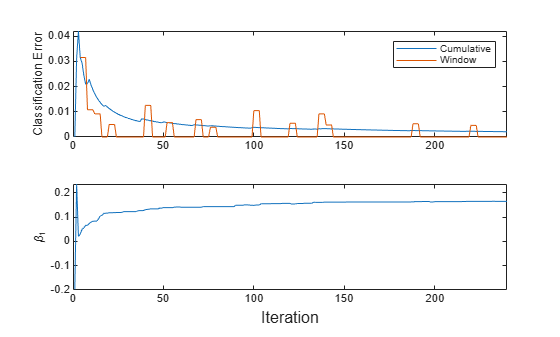# Implement Incremental Learning for Classification Using Flexible Workflow

This example shows how to use the flexible workflow to implement incremental learning for binary classification with prequential evaluation. A traditionally trained model initializes the incremental model. Specifically, this example does the following:

1. Train a linear model for binary classification on a subset of data.

2. Convert the traditionally trained model to an incremental learning model for binary classification.

3. Simulate a data stream using a for loop, which feeds small chunks of observations to the incremental learning algorithm.

4. For each chunk, use updateMetrics to measure the model performance given the incoming data, and then use fit to fit the model to that data.

Although this example treats the application as a binary classification problem, you can implement multiclass incremental learning using an object for a multiclass problem by following this same workflow.

Load the human activity data set. Randomly shuffle the data. Orient the observations of the predictor data in columns.

load humanactivity rng(1) % For reproducibility n = numel(actid); idx = randsample(n,n); X = feat(idx,:)'; Y = actid(idx);

For details on the data set, enter Description at the command line.

Responses can be one of five classes: Sitting, Standing, Walking, Running, or Dancing. Dichotomize the response by identifying whether the subject is moving (actid > 2).

Y = Y > 2;

### Train Linear Model for Binary Classification

Fit a linear model for binary classification to a random sample of half the data. Specify that the observations are oriented along the columns of the data.

idxtt = randsample([true false],n,true); TTMdl = fitclinear(X(:,idxtt),Y(idxtt),'ObservationsIn','columns')
TTMdl = ClassificationLinear ResponseName: 'Y' ClassNames: [0 1] ScoreTransform: 'none' Beta: [60x1 double] Bias: -0.3965 Lambda: 8.2967e-05 Learner: 'svm' Properties, Methods 

TTMdl is a ClassificationLinear model object representing a traditionally trained linear model for binary classification.

### Convert Trained Model

Convert the traditionally trained classification model to a binary classification linear model for incremental learning.

IncrementalMdl = incrementalLearner(TTMdl)
IncrementalMdl = incrementalClassificationLinear IsWarm: 1 Metrics: [1x2 table] ClassNames: [0 1] ScoreTransform: 'none' Beta: [60x1 double] Bias: -0.3965 Learner: 'svm' Properties, Methods 

### Implement Incremental Learning

Use the flexible workflow to update model performance metrics and fit the incremental model to the training data by calling the updateMetrics and fit functions separately. Simulate a data stream by processing 50 observations at a time. At each iteration:

1. Call updateMetrics to update the cumulative and window classification error of the model given the incoming chunk of observations. Overwrite the previous incremental model to update the losses in the Metrics property. Note that the function does not fit the model to the chunk of data—the chunk is "new" data for the model. Specify that the observations are oriented in columns.

2. Call fit to fit the model to the incoming chunk of observations. Overwrite the previous incremental model to update the model parameters. Specify that the observations are oriented in columns.

3. Store the classification error and first estimated coefficient ${\beta }_{1}$.

% Preallocation idxil = ~idxtt; nil = sum(idxil); numObsPerChunk = 50; nchunk = floor(nil/numObsPerChunk); ce = array2table(zeros(nchunk,2),'VariableNames',["Cumulative" "Window"]); beta1 = [IncrementalMdl.Beta(1); zeros(nchunk,1)]; Xil = X(:,idxil); Yil = Y(idxil); % Incremental fitting for j = 1:nchunk ibegin = min(nil,numObsPerChunk*(j-1) + 1); iend = min(nil,numObsPerChunk*j); idx = ibegin:iend; IncrementalMdl = updateMetrics(IncrementalMdl,Xil(:,idx),Yil(idx),... 'ObservationsIn','columns'); ce{j,:} = IncrementalMdl.Metrics{"ClassificationError",:}; IncrementalMdl = fit(IncrementalMdl,Xil(:,idx),Yil(idx),'ObservationsIn','columns'); beta1(j + 1) = IncrementalMdl.Beta(end); end

IncrementalMdl is an incrementalClassificationLinear model object trained on all the data in the stream.

Alternatively, you can use updateMetricsAndFit to update performance metrics of the model given a new chunk of data, and then fit the model to the data.

### Inspect Model Evolution

Plot a trace plot of the performance metrics and estimated coefficient ${\beta }_{1}$.

t = tiledlayout(2,1); nexttile h = plot(ce.Variables); xlim([0 nchunk]) ylabel('Classification Error') legend(h,ce.Properties.VariableNames) nexttile plot(beta1) ylabel('\beta_1') xlim([0 nchunk]) xlabel(t,'Iteration')The cumulative loss is stable and decreases gradually, whereas the window loss jumps.

${\beta }_{1}$ changes abruptly at first, and then gradually levels off as fit processes more chunks of observations.